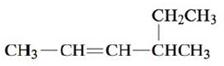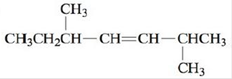# Name each of the following alkenes. a. CH2 = CH — CH 2 — CH 3 b. c.### Chemistry: An Atoms First Approach

2nd Edition
Steven S. Zumdahl + 1 other
Publisher: Cengage Learning
ISBN: 9781305079243

#### Solutions

Chapter
Section### Chemistry: An Atoms First Approach

2nd Edition
Steven S. Zumdahl + 1 other
Publisher: Cengage Learning
ISBN: 9781305079243
Chapter 21, Problem 25E
Textbook Problem
3 views

## Name each of the following alkenes.a. CH2 = CH — CH2 — CH3b.c.(a)

Interpretation Introduction

Interpretation: The given alkenes are to be named.

Concept introduction: Rules given by IUPAC should be followed to name an organic compound. Any organic compound that has only one name denotes that compound. The root word determines the number of carbons while counting the longest carbon chain. Double of triple bond should be given lowest carbon number. If more than one substituent are present, prefixes like di, tri, tetra, etc. are used.

To determine: The name of the given alkene.

### Explanation of Solution

Explanation

The given compound is,

Figure 1

Since, there are four carbons present...

(b)

Interpretation Introduction

Interpretation: The given alkenes are to be named.

Concept introduction: Rules given by IUPAC should be followed to name an organic compound. Any organic compound that has only one name denotes that compound. The root word determines the number of carbons while counting the longest carbon chain. Double of triple bond should be given lowest carbon number. If more than one substituent are present, prefixes like di, tri, tetra, etc. are used.

To determine: The name of the given alkene.

(c)

Interpretation Introduction

Interpretation: The given alkenes are to be named.

Concept introduction: Rules given by IUPAC should be followed to name an organic compound. Any organic compound that has only one name denotes that compound. The root word determines the number of carbons while counting the longest carbon chain. Double of triple bond should be given lowest carbon number. If more than one substituent are present, prefixes like di, tri, tetra, etc. are used.

To determine: The name of the given alkene.

### Still sussing out bartleby?

Check out a sample textbook solution.

See a sample solution

#### The Solution to Your Study Problems

Bartleby provides explanations to thousands of textbook problems written by our experts, many with advanced degrees!

Get Started

Find more solutions based on key concepts
Becoming a vegan takes a strong commitment and significant education to know how to combine foods and in what q...

Nutrition: Concepts and Controversies - Standalone book (MindTap Course List)

16-9 In what way are pyridine and pyrimidine related to benzene?

Introduction to General, Organic and Biochemistry

What is the hybridization of nitrogen in

Chemistry: Principles and Reactions

What is oxyhemoglobin? Where does it form?

Human Biology (MindTap Course List)

Why is there a lower limit to the mass of a main-sequence star?

Horizons: Exploring the Universe (MindTap Course List)

A thermodynamic process occurs in which the entropy of a system changes by 8 J/K. According to the second law o...

Physics for Scientists and Engineers, Technology Update (No access codes included)

What are possible fates (or end-states) for comets?

Foundations of Astronomy (MindTap Course List)